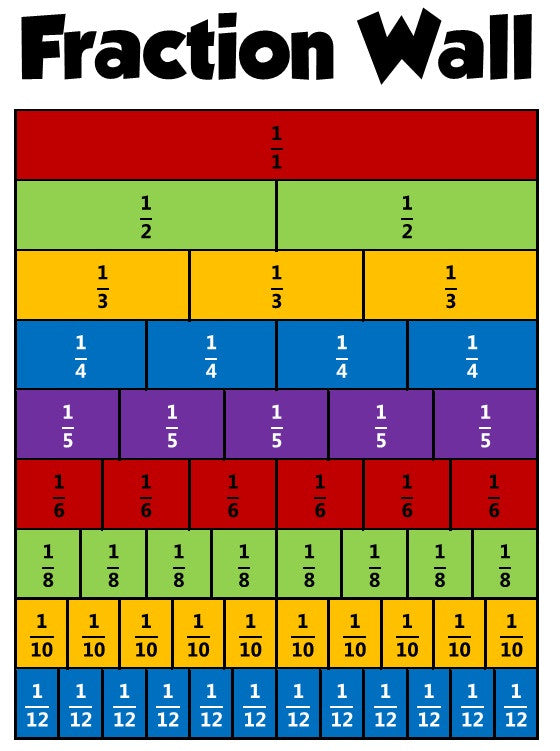Bringing Singapore Education Worldwide
We ship from Australia

# Singapore Books News — Fractions

## Why are fractions so difficult to learn?

Fractions MathsAs many teachers and parents know, learning the various fraction operations can be difficult for many children. It's not the concept of a fraction that is difficult - it is the various operations: addition, subtraction, multiplication, division, comparing, simplifying, etc. of fractions And the simple reason why learning those operations proves difficult for many students is the way they are typically taught. Just look at the amount of rules there are to learn about fractions! 1. Fraction addition - common denominators Add the numerators, and use the common denominator 2. Fraction addition - different denominators First find a common denominator...